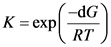# What is the value of K for this aqueous reaction at 298 K? Delta G= 12.88 Kj/mol

What is the value of K for this aqueous reaction at 298 K? Delta G= 12.88 Kj/mol

A+B<–> C+D

Concepts and reason
The concept used to solve this problem is based on the calculation of equilibrium constant by using change in Gibbs free energy.

To calculate maximum reversible work done, thermodynamic potential used at constant pressure and temperature is called Gibbs free energy.

In a chemical reaction, the value of reaction quotient when the reaction reach equilibrium is called equilibrium constant.

Fundamentals
The relation between change in Gibbs free energy and equilibrium constant is as follow,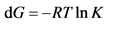Here dG is change in Gibbs free energy,T is temperature at which reaction takes place, K is equilibrium constant and R is gas constant.

Convert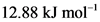of Gibbs free energy change into joules.Explanation:
Since one kilojoule is equal to 1000 joules. So,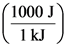is used as a conversion factor.

Rearrange the formula for Gibbs free energy change.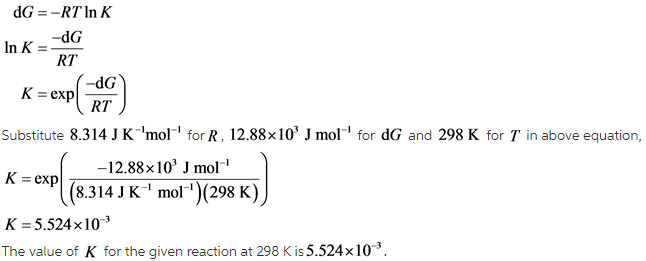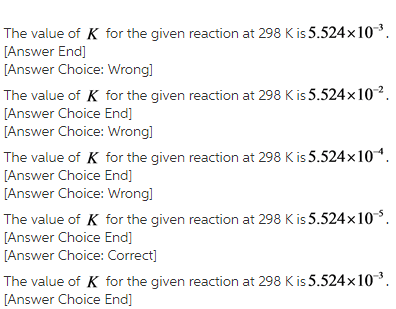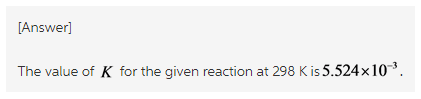Explanation:
The equilibrium constant is calculated by rearranging the formula of the Gibbs free energy change as follow.